# Scatter Plots in Python

Scatterplots are one of many crucial forms of visualization in statistics. With scatterplots, you can examine the relationship between two variables. This can lead to insights in terms of decision making or additional analysis.We will be using the “Prestige” dataset form the pydataset module to look at scatterplot use. Below is some initial code.

```from pydataset import data
import matplotlib.pyplot as plt
import pandas as pd
import seaborn as sns
df=data('Prestige')```

We will begin by making a correlation matrix. this will help us to determine which pairs of variables have strong relationships with each other. This will be done with the .corr() function. below is the code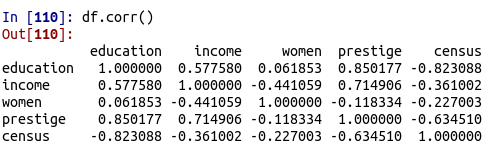You can see that there are several strong relationships. For our purposes, we will look at the relationship between education and income.

The seaborn library is rather easy to use for making visuals. To make a plot you can use the .lmplot() function. Below is a basic scatterplot of our data.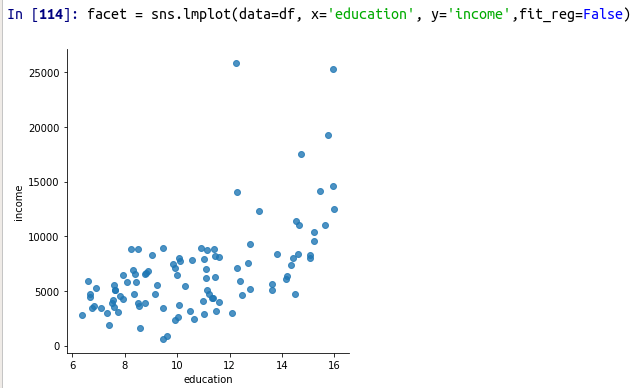The code should be self-explanatory. THe only thing that might be unknown is the fit_reg argument. This is set to False so that the function does not make a regression line. Below is the same visual but this time with the regression line.

`facet = sns.lmplot(data=df, x='education', y='income',fit_reg=True)`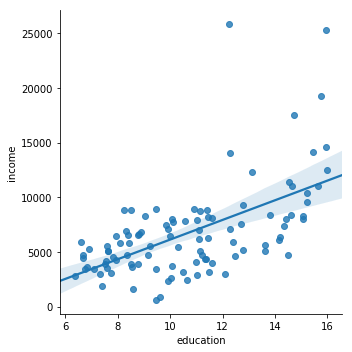It is also possible to add a third variable to our plot. One of the more common ways is through including a categorical variable. Therefore, we will look at job type and see what the relationship is. To do this we use the same .lmplot.() function but include several additional arguments. These include the hue and the indication of a legend. Below is the code and output.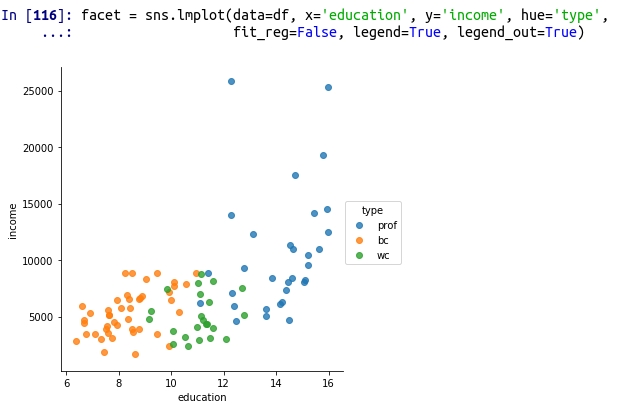You can clearly see that type separates education and income. A look at the boxplots for these variables confirms this.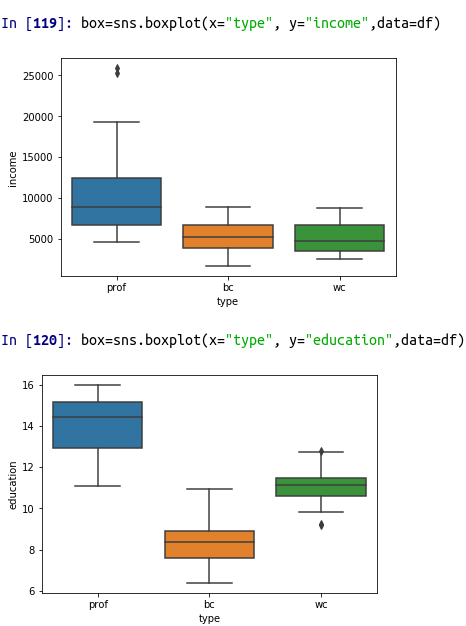As you can see, we can conclude that job type influences both education and income in this example.

Conclusion

This post focused primarily on making scatterplots with the seaborn package. Scatterplots are a tool that all data analyst should be familiar with as it can be used to communicate information to people who must make decisions.